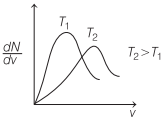# Degrees of Freedom in Physics | Definition, Formula – Kinetic Theory of Gases

Degrees of Freedom in Physics Definition:
The degree of freedom for a dynamic system is the number of directions in which a particle can move freely or the total number of coordinates required to describe completely the position and configuration of the system.
It is denoted by f or N.

We are giving a detailed and clear sheet on all Physics Notes that are very useful to understand the Basic Physics Concepts.

## Degrees of Freedom in Physics | Definition, Formula – Kinetic Theory of Gases

Degrees of Freedom Formula Physics:
Suppose if we have A number of gas molecules in the container, then the total number of degrees of freedom is f = 3A. But, if the system has R number of constraints (restrictions in motion) then the degrees of freedom decreases and it is equal to f = 3A-R where A is the number of particles.
Degree of freedom of a system is given by

f or N = 3A – R

where,
A = number of particles in the system and
R = number of independent relations between the particles.

Degree of freedom for different atomic particles are given below.

1. For monoatomic gas = 3 (all translational).
2. For diatomic gas = 5 (3 translational, 2 rotational)
3. For non-linear triatomic gas = 6 (3 translational, 3 rotational)
4. For linear triatomic gas = 7 (3 translational,3 rotational and 1 vibrational)

Specific heat of a gas

(a) At constant volume, Cv = $$\frac{f}{2}$$R.
(b) At constant pressure, Cp = $$\left(\frac{f}{2}+1\right)$$R
(c) Ratio of specific heats of a gas at constant pressure and at constant volume is given by γ = 1 + $$\frac{2}{f}$$

Specific heat of solids, C = 3R ⇒ C = 24.93 Jmol-1 K-1.
Specific heat of water, C = 9R ⇒ C = 74.97 Jmol-1 K-1.Maxwell’s Law or the Distribution of Molecular Speeds
It derives an equation giving the distribution of molecules at different speeds

dN = 4πN$$\left(\frac{m}{2 \pi k T}\right)^{3 / 2} v^{2} e^{-\left(\frac{m v^{2}}{2 k T}\right)} \cdot d v$$

where, dN is number of molecules with speed between v and v + dv.
The $$\frac{d N}{d v}$$ versus v curve is shown below### Law of Equipartition of Energy

This law states that, for a dynamic system in thermal equilibrium, the total energy is distributed equally amongst all the degree of freedom and the energy associated with each molecule per degree of freedom is

$$\frac{1}{2}$$ kB T.

where,
kB = 1.38 x 10-23 JK-1 is Boltzmann constant and
T is the absolute temperature of system on the kelvin scale.

Kinetic Theory of Gases:
In this concept, it is assumed that the molecules of gas are very minute with respect to their distances from each other. The molecules in gases are in constant, random motion and frequently collide with each other and with the walls of any container.

In this portion, you will learn about the properties of gases, based on density, pressure, temperature and energy. Continue reading here to learn more.